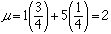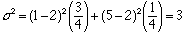Subject: RE: A note on Standard Deviation Name: Jonathan Who is asking: Teacher Level: Secondary Question: I was just reading your article entitled A Note on Standard Deviation. I'm now teaching a unit on s.d. and my students were wondering why one uses a denominator of n for a population and n-1 for a sample. I saw in your article that this is because "[the quantity] tends to underestimate sigma... and other technical reasons." To which my students again asked... "Why?" Could you please elaborate a bit on the "other technical reasons" perhaps in terms a high school senior (or their teacher...) could understand? Thank you for your time. Jonathan Hi Jonathan, Rather than trying to give a theoretical elaboration on what I called "other technical reasons" I want to suggest an experiment you can try with your class that should help illustrate how "[the quantity] tends to underestimate sigma" and indicate one of the "other technical reasons". I am actually going to work with sigma squared, the variance, rather than sigma. I am going to describe the experiment in terms of white beans and black bean. You can use any items that you can find that are indistinguishable by feel but of two different kinds visually. You need somewhere between 60 and 100 items with 1/4 of one kind "white beans" and 3/4 of the other "black beans". Give each white bean a value of 5 and each black bean a value of 1. This is the population. The proportions as well as the mean and variance of the population should be kept unknown to your students until the end of the experiment. The mean of the population values isand the variance isThe task for the students is to estimate the mean and the variance of the values of the beans using a random sample. To keep the arithmetic easy I suggest that you use a sample size of n = 3. Put the beans in a bag or jar where the students can't see inside and have them, one at a time select a random sample of size 3 and record the number of white and black beans in the sample. The student then replaces the beans before the next student selects his or her sample. It would be best if you had 50 to 60 samples so you may want each student to select two samples and deal with each independently. Each student should then compute the mean and variance of his or her sample values. Have them calculate the variance twice, once dividing by n and once by n-1. Now have the students report their results to you and you should record them in 3 columns, one column of means, one column of variances where the students divided by n and the last column, variances where the students divided by n - 1. You will see that the mean column has only 4 different numbers in it and each of the other two columns have only 2 different numbers in them. This is caused by the sample size, n = 3, being so small. If the sample size were large there would be more values in each column. Concentrate first on the column of means. Each item in this column is an estimate of the population mean. Now tell them the population mean and they will see that some estimates are too large and some are two small. Finally calculate the average of the 50 or so items in the "mean" column. This average should be quite close to 2, the population mean. The theory says that "on the average" the sample mean is the same as the population mean. The name given to this property is unbiased, the sample mean is an unbiased estimator for the population mean. Now consider the second and third columns, find their means and tell the class the population variance. The average of the variance column where you divided by n - 1 should be quite close to the population variance while the average of the other column will be considerably smaller. The sample variance (dividing by n - 1) is an unbiased estimator for the population variance. The sample variance (dividing by n) is a biased estimator, "tends to underestimate sigma". One last point. A denominator of n - 1 is used when computing the sample variance as it yields an unbiased estimator for the population variance. Might there however be another estimator for the population variance that would be preferred over the sample variance? Not only would you want this new estimator to be unbiased but it would be nice if most of the values in the column for the new estimator were close to the population variance. That is you want the variability in the column of values of the new estimator to be small. This is one of the "other technical reasons" why the sample variance is used to estimate the population variance. Of all the reasonable estimators you could use, the sample variance has the smallest variability. I hope that this helps. If you have your class do the experiment I suggested, let me know how it turns out. Cheers, Harley Go to Math Central